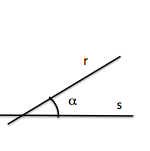# Metric geometry: Notions of anglesGeometric elements in the plane intersecting, lines and circles, can characterize its intersection by a value called angle.

The notion of angle between two lines is the most basic among those between lines and circles, and serves as a reference to define the angle between line and circle or two circles forming coplanar.

To define an angle we must remember that a line r dividing plane into two half-planes.

A new line s divide the plane into two new half-planes, whose intersection with the above four regions determine infinite area, but equal in pairs.

There are three measurement systems angles:

• Radians : Values ​​between 0 and twice the value of PI
• Sexagesimal: Values ​​between zero and 360
• Centesimal: Values ​​between zero and 400

## Angle between two lines

Angle concept is used to measure or characterize an infinite area, a portion of the plane which in turn is the intersection of two half-planes.

An angle is the plane between two rays that have the same point of origin or vertex. Usually measured in units such as the radian, sexagesimal degree or grad. (W)

The first diero definitions of these concepts are called classical definitions:

Euclid defines an angle as the mutual inclination of two lines which meet one another in a plane and are not in a straight line. By Proclus, angle must be a quality or a quantity, or a relationship. The first concept was used by Eudemus of Rhodes, which described an angle as a deviation from a straight line; the second by Carpus of Antioch, saw it as the interval or space between the intersecting lines; Euclid adopted the third concept, although his definitions of right angles, acute, and obtuse are quantitative. (W)

The value of angle between two lines always given as the smaller of the two that determine.

## Angle between line and circle

To define the angle between a line and a circle incidents, determine the tangent at the intersection point.

We will reduce the problem of two lines.

The angle between a line and a circle are cut, is between the line tangent to the circumference on one of the intersection points.

The line can be cut at two points to the circumference; If we calculate the angle at any point of intersection, the value is the same.

## Angle between two circles

To define the angle between two incident circumference, determine one of the tangents at the points of intersection.

We will reduce the problem of two lines.

The angle between two circles which intersect, is formed by its tangent at any of their points of intersection.

The circles can be cut at two points; If we calculate the angle at any point of intersection, the value is the same.

Metric geometry